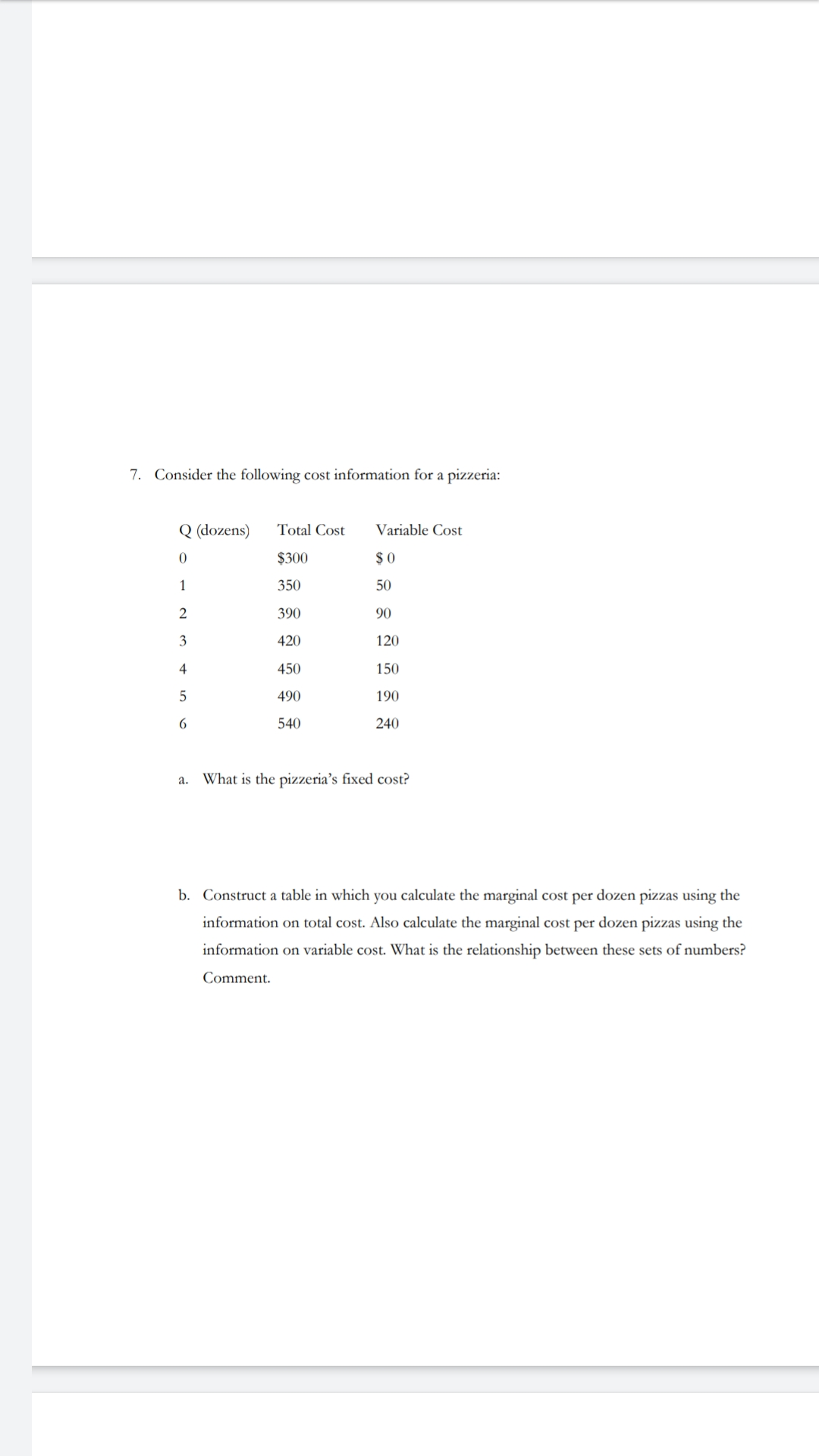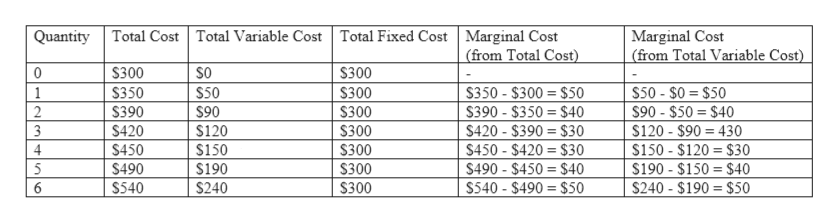# 7. Consider the following cost information for a pizzeria:Q (dozens)Variable CostTotal Cost\$ 0\$3003505012390904201204501504901905406240What is the pizzeria's fixed cost?а.b. Construct a table in which you calculate the marginal cost per dozen pizzas using theinformation on total cost. Also calculate the marginal cost per dozen pizzas using theinformation on variable cost. What is the relationship between these sets of numbers?Comment

Question
26 viewshelp_outlineImage Transcriptionclose7. Consider the following cost information for a pizzeria: Q (dozens) Variable Cost Total Cost \$ 0 \$300 350 50 1 2 390 90 420 120 450 150 490 190 540 6 240 What is the pizzeria's fixed cost? а. b. Construct a table in which you calculate the marginal cost per dozen pizzas using the information on total cost. Also calculate the marginal cost per dozen pizzas using the information on variable cost. What is the relationship between these sets of numbers? Comment fullscreen
check_circle

Step 1

The fixed costs are the costs that are incurred even if there is no production. These include the rent paid, the capital employed etc. These costs always exists and remain constant irrespective of the level of output.

Step 2

It is given that when the firm is producing zero units of output then the total costs are equal to \$300. The total costs are the sum of the total fixed costs and the total variable costs. Since the variable costs are zero at that level of output, the entire cost is the fixed cost. So, the total fixed costs are \$300.

Step 3

The marginal cost is the additional cost incurred by the firm when one more unit of output is produced. It can be calculated by the difference in th...help_outlineImage TranscriptioncloseTotal Cost Total Variable Cost Total Fixed Cost Marginal Cost (from Total Cost) Quantity Marginal Cost (from Total Variable Cost) \$300 \$300 \$50-\$0 = \$50 \$90-\$50 = \$40 \$120- \$90 430 \$150- \$120 \$30 \$190-\$150 \$40 \$240-\$190 \$50 S350-\$300= \$50 \$390-\$350 = \$40 \$420- \$390 = \$30 \$450 - \$420 \$30 \$490-\$450 = \$40 \$540-\$490 = \$50 1 \$350 \$50 \$90 \$300 2 \$390 \$300 \$420 S450 \$490 \$120 \$300 4 5 \$150 \$300 \$190 \$300 \$240 \$540 \$300 fullscreen

### Want to see the full answer?

See Solution

#### Want to see this answer and more?

Solutions are written by subject experts who are available 24/7. Questions are typically answered within 1 hour.*

See Solution
*Response times may vary by subject and question.
Tagged in